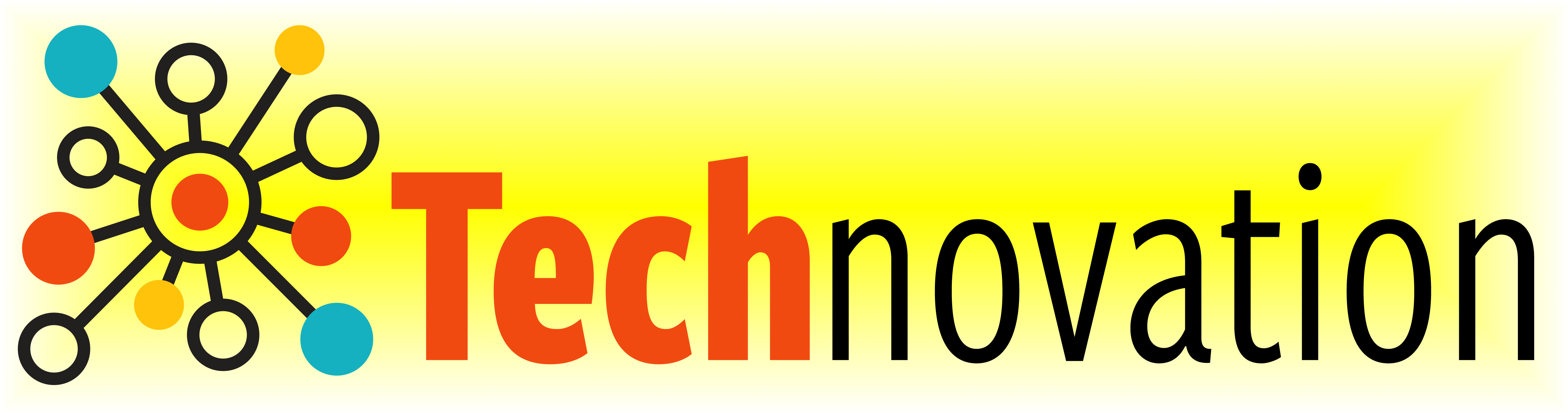# 6.5. Practice Makes Perfect¶

Here’s a reminder of the drawing commands we’ve learned so far:

Drawing commands

What does it do?

`forward( distance )`

Move forward a specified distance

`backward( distance )`

Move backward a specified distance

`left(90)`

Turns 90 degrees to the left (you can use any angle, not just 90!)

`right(90)`

Turns 90 degrees to the right

`circle( radius )`

Draws a circle with the specified radius

`goto( x, y )`

Move straight to the position with coordinates (x, y). Note: the center is (0, 0)

`up()`

Stop leaving a trail

`down()`

Start drawing a trail

`color( c )`

Set the color to c (https://trinket.io/docs/colors)

`begin_fill()`

Starts filling in drawn shapes

`end_fill()`

Stops filling in drawn shapes

`speed(number 0-10)`

Determines how quickly the turtle will move through commands

Functions and Loops

Drawing commands

What does it do?

`for i in range( n ):`

Repeat n times

`def function_name():`

Creates and defines a new function

`function_name()`

Calls a function

And here are the new commands we’ll be learning this week!

Command

What does it do?

`print( message )`

Output the message

`variable = value`

Stores the value in your variable

`variable = input( message )`

Takes user input and stores it in your variable

What are variables and user input all about?

Let’s test out how to use variables! Use the code below or python tutor to walk through some examples.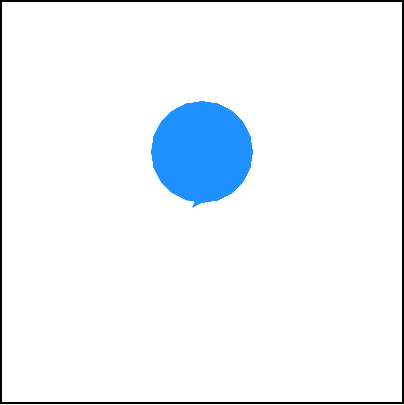How could you use variables and user input to draw different colored circles?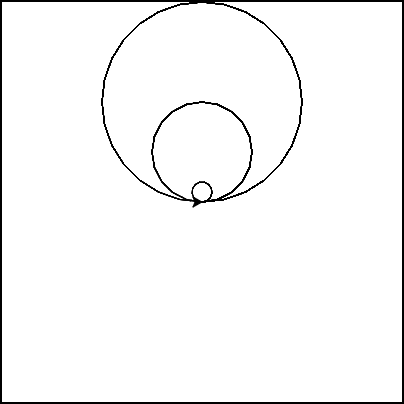How could you use variables and user input to draw different sized circles? Run the code below to see how you can use variables with turtle commands. Try changing the radius variable to see what it does!
Then try drawing a third cirlce that gets the radius value from the user!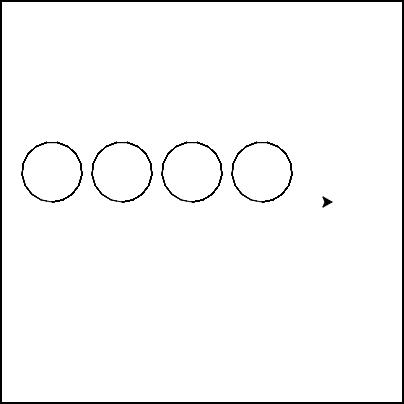How could we repeat code as many times as the user wants?
Let’s combine what we know about user input with what we know about loops!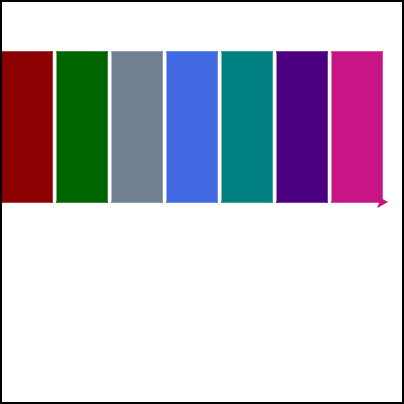How could you use variables and user input to draw a library?
Every library must have books - could you define a function to draw a book?
Then, could you take user input to set the color of each book?
Give it a try!